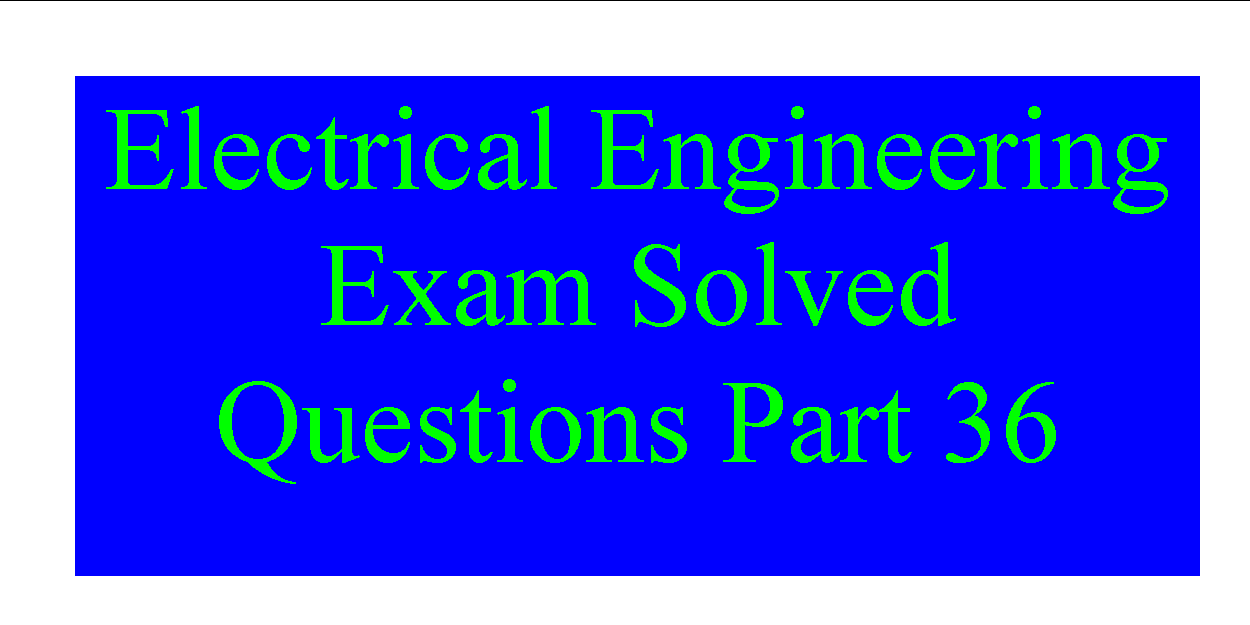## Electrical Engineering Exam Solved Questions Part 36

Electrical Engineering Solved Questions published below these are helpful for preparing upcoming EE JEE SEE Recruitment Examinations. Also study current affairs as 5-15 Current affairs questions included in the examination
Electrical Engineering Question No # 5011. The number of brushes in a commutator depends on
(a) speed of armature
(b) type of winding
(c) voltage
(d) amount of current to be collected

Answer: (d) amount of current to be collected

Electrial Engineering Question No # 5012. The high voltage winding is usually which of the following type ?
(a) Cylindrical winding with circular conductors
(b) Cross-over winding with either circular or small rectangular conductors
(c) Continuous disc type winding with rectangular conductors
(d) All of the above types
Ans: dElectrical Engineering Question No # 5013. What is the minimum number of conversions per second of a clock rate of 1 MHz operating a 10-
A) 1000
B) 976
C) 769
D) 697

Electrical Engineering Question No # 5014. Tractive magnets are operated from
(a) a.c. supply
(b) d.c. supply
(c) either a.c. or d.c. supply
(d) none of the above
Ans: c

### GK Questions- Listed here

Electrical Engineering Question No # 5015. The armature of D.C. generator is laminated to
(a) reduce the bulk
(b) provide the bulk
(c) insulate the core
(d) reduce eddy current loss

Answer: (d) reduce eddy current loss

Electri cal Engineering Question No # 5016. In an alternator if the winding is short pitched by 50 electrical degrees, its pitch factor will be

(A) 1.0

(B) 0.866

(C) 0.75

(D) 0.50.

Electrical Engineering Question No # 5017. In a D.C. machine, the value of peripheral speed should not, normally, exceed
(a) 10 m/s
(b) 20 m/s
(c) 30 m/s
(d) 40 m/s
Ans: cElectrical Engineering Question No # 5018. The main difference between the isolation amplifier and an instrumentation amplifier is
A) an instrumentation amplifier has an input stage.
B) an instrumentation amplifier has an output stage.
C) an instrumentation amplifier has a differential stage
D) the input, output, and power supply stages of an isolation amplifier are all electrically isolated from each other
Electrical Engineering Question No # 5019. In lap winding, the number of brushes is always
(a) double the number of poles
(b) same as the number of poles
(c) half the number of poles
(d) twoAnswer: (b) same as the number of polesElectrical Engineering Question No # 5020. Equilizer rings are required in case armature is
(a) wave wound
(b) lap wound
(c) delta wound
(d) duplex woundAnswer: (b) lap wound

More Engineering Jobs– Listed Below